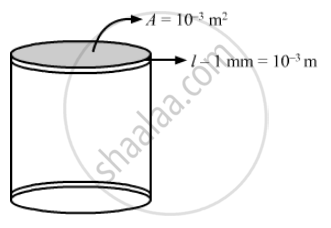Share

# Water at 50°C is Filled in a Closed Cylindrical Vessel of Height 10 Cm and Cross Sectional Area 10 Cm2. the Walls of the Vessel Are Adiabatic but the Flat Parts Are Made of 1-mm Thick Aluminium - Physics

ConceptThermal Expansion of Solids

#### Question

Water at 50°C is filled in a closed cylindrical vessel of height 10 cm and cross sectional area 10 cm2. The walls of the vessel are adiabatic but the flat parts are made of 1-mm thick aluminium (K = 200 J s−1 m−1°C−1). Assume that the outside temperature is 20°C. The density of water is 100 kg m−3, and the specific heat capacity of water = 4200 J k−1g °C−1. Estimate the time taken for the temperature of fall by 1.0 °C. Make any simplifying assumptions you need but specify them.

#### Solution

Area of cross section, A = 10 cm2 = 10 × 10–4 m2

Thermal conductivity, K = 200 Js–1 m–1 °C–1

Height, H = 10 cm

Length, l = 1 mm =10–3 m

Temperature inside the cylindrical vesselT1 = 50°C

temperature outside the vessel, T2 = 30°CRate of flow of heat from 1 flat surface will be given by

(DeltaQ)/(Deltat) = (T_1 -T_2)/( l /(KA)

(DeltaQ)/(Deltat) = ((50 - 30 ) xx 200 xx 10^-3)/ 10^-3

(DeltaQ)/(Deltat) = 6000 J/s

Heat escapes out from both the flat surfaces.
Net rate of heat flow = 2 × 6000 = 12000 J/sec

(DeltaQ)/(Deltat) = (m.s.DeltaT)/ (Deltat)

Mass = Volume density

⇒10^-3 xx 0.1 xx 1000 =0.1g

Using this in the above formula for finding the rate of flow of heat, we get

12000 = 0.1 xx 4200 xx (DeltaT)/(t)

⇒ (DeltaT)/(Deltat) = 12000/420 = 28.57

As DeltaT = 1^circ C

⇒ 1/t = 28.57

⇒ t = 1/28.57 = 0.035 sec

Is there an error in this question or solution?

#### APPEARS IN

Solution Water at 50°C is Filled in a Closed Cylindrical Vessel of Height 10 Cm and Cross Sectional Area 10 Cm2. the Walls of the Vessel Are Adiabatic but the Flat Parts Are Made of 1-mm Thick Aluminium Concept: Thermal Expansion of Solids.
S